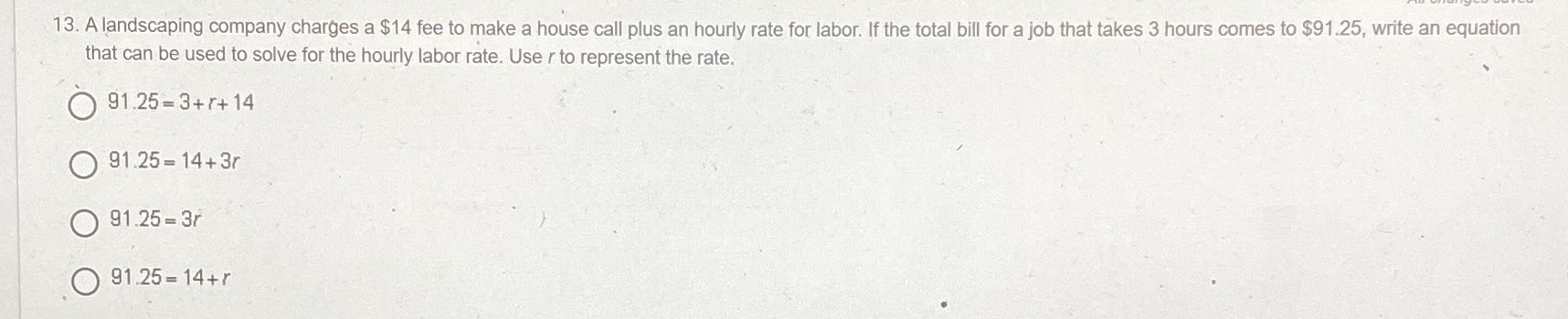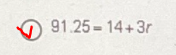### ¿Todavía tienes preguntas de matemáticas?

Pregunte a nuestros tutores expertos
Algebra
PreguntaA landscaping company charges a $$\ 14$$ fee to make a house call plus an hourly rate for labor. If the total bill for a job that takes $$3$$ hours comes to $$\ 91.25 ,$$ write an equation that can be used to solve for the hourly labor rate. Use $$r$$ to represent the rate.

$$91.25 = 3 + r + 14$$

$$91.25 = 14 + 3 r$$

$$91.25 = 3 r$$

$$91.25 = 14 + r$$